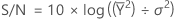# What is the signal-to-noise ratio in a Taguchi design?

In Taguchi designs, a measure of robustness used to identify control factors that reduce variability in a product or process by minimizing the effects of uncontrollable factors (noise factors). Control factors are those design and process parameters that can be controlled. Noise factors cannot be controlled during production or product use, but can be controlled during experimentation. In a Taguchi designed experiment, you manipulate noise factors to force variability to occur and from the results, identify optimal control factor settings that make the process or product robust, or resistant to variation from the noise factors. Higher values of the signal-to-noise ratio (S/N) identify control factor settings that minimize the effects of the noise factors.

Taguchi experiments often use a 2-step optimization process. In step 1 use the signal-to-noise ratio to identify those control factors that reduce variability. In step 2, identify control factors that move the mean to target and have a small or no effect on the signal-to-noise ratio.

The signal-to-noise ratio measures how the response varies relative to the nominal or target value under different noise conditions. You can choose from different signal-to-noise ratios, depending on the goal of your experiment. For static designs, Minitab offers four signal-to-noise ratios:
Signal-to-noise ratio Goal of the experiment Data characteristics Signal-to-noise ratio formulas
Larger is better Maximize the response Positive S/N = −10 *log(Σ(1/Y2)/n)
Nominal is best Target the response and you want to base the signal-to-noise ratio on standard deviations only Positive, zero, or negative S/N = −10 *log(σ2)
Nominal is best (default) Target the response and you want to base the signal-to-noise ratio on means and standard deviations Non-negative with an "absolute zero" in which the standard deviation is zero when the mean is zero